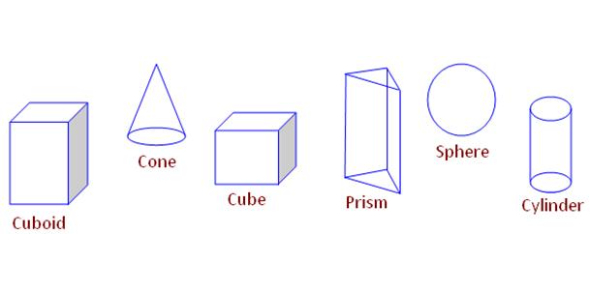# Geometrical Figures: 3rd Grade Quiz!

17 Questions | Attempts: 582Settings.

• 1.
How many edges do a kite have?
• A.

1

• B.

2

• C.

4

• D.

3

• 2.
How many corners does a kite have?
• A.

2

• B.

4

• C.

6

• D.

8

• 3.
How many edges does the table have?
• A.

2

• B.

4

• C.

3

• D.

1

• 4.
How many corners does the surface have?
• A.

1

• B.

2

• C.

3

• D.

4

• 5.
What is the shape of the surface of the table?
• A.

Triangle

• B.

Square

• C.

Rectangle

• D.

Circle

• 6.
How many edges does a rectangle have?
• A.

4

• B.

3

• C.

2

• D.

1

• 7.
How many corners does a rectangle have?
• A.

2

• B.

3

• C.

1

• D.

4

• 8.
Does a circle have edges?
• A.

Yes

• B.

No

• 9.
Does a circle have a corner?
• A.

Yes

• B.

No

• 10.
How many edges does a triangle have?
• A.

4

• B.

2

• C.

6

• D.

3

• 11.
How many corners does a triangle have?
• A.

1

• B.

2

• C.

5

• D.

3

• 12.
Fill in the blanks.  circle have .......edges and corners
• A.

2

• B.

3

• C.

4

• D.

0

• 13.
Triangle has........edges.
• A.

2

• B.

4

• C.

3

• D.

6

• 14.
Square have ........ corners.
• A.

4

• B.

6

• C.

8

• D.

5

• 15.
Rectangle has........ corners.
• A.

4

• B.

5

• C.

8

• D.

6

• 16.
Triangle has......Corners.
• A.

9

• B.

6

• C.

3

• D.

5

## Related TopicsBack to top
×

Wait!
Here's an interesting quiz for you.